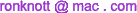This page is about the trigonometric functions of sine, cosine and tangent, what they are and how to find the exact values of many angles.
The calculators and other effects on this page require JavaScript but you appear to have switched JavaScript off (it is disabled) in this browser.

# Exact Trigonometric Function Values

What angles have an exact expression for their sines, cosines and tangents? You might know that cos(60°)=1/2 and sin(60°)=√3/2 as well as tan(45°)=1, but are 30, 45 and 60 the only angles up to 90° with a formula for their trig values? No! There are lots more but not all angles have exact expressions involving nothing more than square-roots.
Which angles do? What patterns are there in these expressions? This page shows expressions for many angles and even solves the complete problem of which angles do and which don't have such exact trig expressions.

## A Table of Exact Trig values

that are expressible as simple terms involving square-roots.

a cos(a)
sin(b)
tan(a)
cot(b)
b
0 01 0 90 π
2
π
24
7.5 color{blue}{(sqrt(2+sqrt(2+sqrt 3)))/2} color{blue}{sqrt 6 - sqrt 3 + sqrt 2 - 2}
color{blue}{= (sqrt 2 - 1)(sqrt 3 - sqrt 2)}
82.5 11 π
24
π
12
15 color{blue}{(sqrt 6 + sqrt 2)/4 = sqrt(1/2+(sqrt 3)/4) = sqrt((4+ sqrt 12)/8)}
color{blue}{2-sqrt 3}
75 5 π
12
cos2(15°)=[0;1,13, 1,12] tan(15°) = [0; 3, 1, 2 ]
tan2(15°) = [0; 13, 1, 12]
π
10
18 color{blue}{sqrt(10+2 sqrt 5)/4 = sqrt((5+sqrt 5)/8)}
color{blue}{sqrt(1- (2 sqrt 5)/5)} 72 2 π
5
cos2(18°) = [0; 1, 9, 2, 8] tan2(18°) = [0; 9, 2,8]
π
8
22.5 color{blue}{(sqrt(2+sqrt 2))/2 = sqrt((4+sqrt 8)/8)} color{blue}{sqrt 2 - 1} 67.5 3 π
8
cos2(22.5°) = [0; 1, 5, 1, 4] tan(22.5°) = [0; 2]
tan2(22.5°) = [0; 5, 1, 4]
π
6
30 color{blue}{ (sqrt 3)/2} color{blue}{(sqrt 3)/3} 60 π
3
cos(30°) = [ 0; 1, 6, 2, 6 ]
cos2(30°) = [0; 1, 3]
tan(30°) = [ 0; 1, 1, 2 ]
tan2(30°) = [0; 3 ]
π
5
36 color{blue}{(sqrt 5 + 1)/4 = sqrt((3+sqrt 5)/8)} color{blue}{sqrt(5 - 2 sqrt 5)} 54 3 π
10
cos(36°) = [ 0; 1, 4 ]
cos2(36°) = [ 0; 1, 1, 1, 8, 2 ]
tan2(36°) = [ 0; 1, 1, 8, 2 ]
5 π
24
37.5 color{blue}{sqrt(2 + sqrt( 2 - sqrt 3))/2 } color{blue}{sqrt 6 + sqrt 3 - sqrt 2 - 2}
color{blue}{= (sqrt 2 + 1)(sqrt 3 - sqrt 2)}
52.5 7 π
24
π
4
45 color{blue}{sqrt 2/2} color{blue}{1} 45 π
4
cos(45°) = [ 0; 1, 2 ]
cos2(45°) = [ 0; 2 ]
7 π
24
52.5 color{blue}{(sqrt(2 - sqrt( 2 - sqrt 3)))/2} color{blue}{sqrt 6 - sqrt 3 - sqrt 2 + 2}
color{blue}{= (sqrt 2 - 1)(sqrt 3 + sqrt 2)}
37.5 5 π
24
3 π
10
54 color{blue}{(sqrt(10 - 2 sqrt 5))/4 = sqrt((5 - sqrt 5)/8)} color{blue}{sqrt((5 + 2 sqrt 5)/5)} 36 π
5
cos2(54°) = [ 0; 2, 1, 8, 2 ] tan2(54°) = [ 1; 1, 8, 2 ]
π
3
60 color{blue}{1/2} color{blue}{sqrt 3} 30 π
6
cos(60°) = [0; 2]
cos2(60°) = [0; 4]
tan(60°) = [ 1; 1, 2 ]
tan2(60°) = 3
3 π
8
67.5 color{blue}{sqrt(2-sqrt 2)/2 = sqrt((4-sqrt 8)/8)} color{blue}{1 + sqrt 2} 22.5 π
8
cos2(67.5°) = [ 0; 6, 1, 4 ] tan(67.5°) = [ 2; 2 ]
tan2(67.5°) = [ 5; 1, 4 ]
2 π
5
72 color{blue}{(sqrt 5 - 1)/4 = sqrt((3-sqrt 5)/8)} color{blue}{sqrt(5 + 2 sqrt 5)} 18 π
10
cos(72°) = [ 0; 3, 4 ]
cos2(72°) = [ 0; 10, 2, 8 ]
tan2(72°) = [ 9; 2, 8 ]
5 π
12
75 color{blue}{(sqrt 6 - sqrt 2)/4 = sqrt(1/2 - sqrt 3/4) = sqrt((4 - sqrt 12)/8)} color{blue}{2+sqrt 3} 15 π
12
cos2(75°) = [ 0; 14, 1, 12 ] tan(75°) = [ 3; 1, 2 ]
tan2(75°) = [ 13; 1, 12 ]
11 π
24
82.5 color{blue}{sqrt(2 - sqrt(2 + sqrt 3))/2} color{blue}{sqrt 6 + sqrt 3 + sqrt 2 + 2}
color{blue}{= (sqrt 2 + 1)(sqrt 3 + sqrt 2)}
7.5 π
24
π
2
90 color{blue}{0} color{blue}{oo} 0 0
The Continued fraction [a; b, c, d, ...] is a shorthand notation for
 a + 1 b + 1 c + 1 d + ...

and the periodic continued fraction [a; b, c,   d, e,   d, e,   d, e, ...] is written as [ a; b, c, d, e ]
where any number before the semicolon (;) is the whole part and the line over the numbers indicates the part that repeats for ever at the end.
See an Introduction to Continued Fractions.

## All the trig functions in one diagram

Here is a really great Mathematica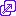demonstration of how all the 6 trigonometric functions are related, in one interactive diagram. Here is a static image which is a useful diagram anyway:

However, the diagram is useful for seeing what is measured by the six functions but it does not give any indication of the signs of the values.
The signs are chosen to make the trig formula see below consistent for all angles. Also, the next section shows their graphs and from the basic SINE graph, all the others follow.
The sine function has many applications in mechanics (e.g. the motions of rotating objects), electronics (e.g. alternating electric current and electromagnetic waves), ... .

### Trig functions of Angles outside the range 0° to 90°

Here are diagrams of an angle in each of the four quadrants of a circle, snapshots from the excellent How the Trigonometry Functions Are Related from the Wolfram Demonstrations Project by C. Ormullion. Click in a quadrant to see a typical angle and all 6 trig functions.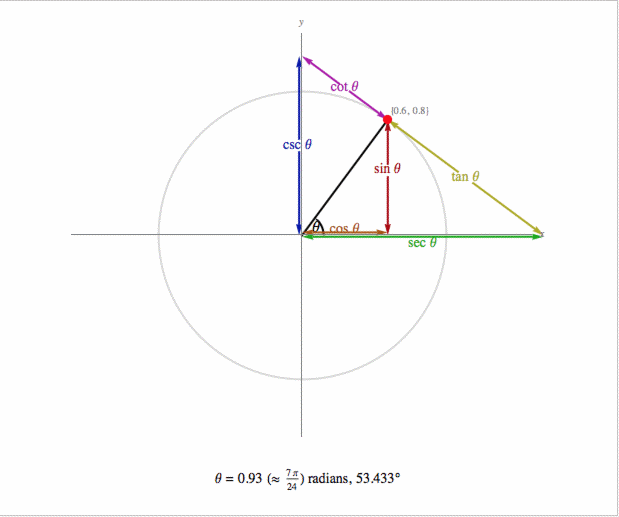Here is another visualization, by graphs: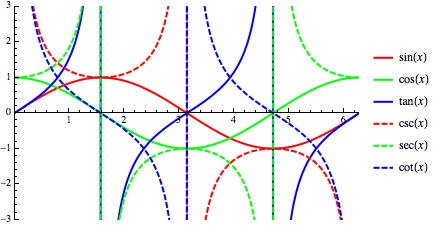A nice way to remember the SIGN to use for the functions in each quadrant is that they are All positive in the first quadrants (0-90°) but in the other quadrants, only one of sin, cos or tan is positive in the other quadrants:
 Sine All Tangent Cosine
You can see that it is written inside a big PLUS (+) sign and the only function(s) that have the plus sign with its values in that quadrant is, in order of the angle side,
"All, Sine, Tangent, Cosine" and you can remember this with the silly rhyme "All Silver Tea Cups".
But why not make up your OWN phrase to remember the letters ASTC?
If it's silly then you are more likely to remember it!
Once you remember the sign for these three functions, you can then use the same sign for their reciprocal values Cosecant, Secant and Cotangent.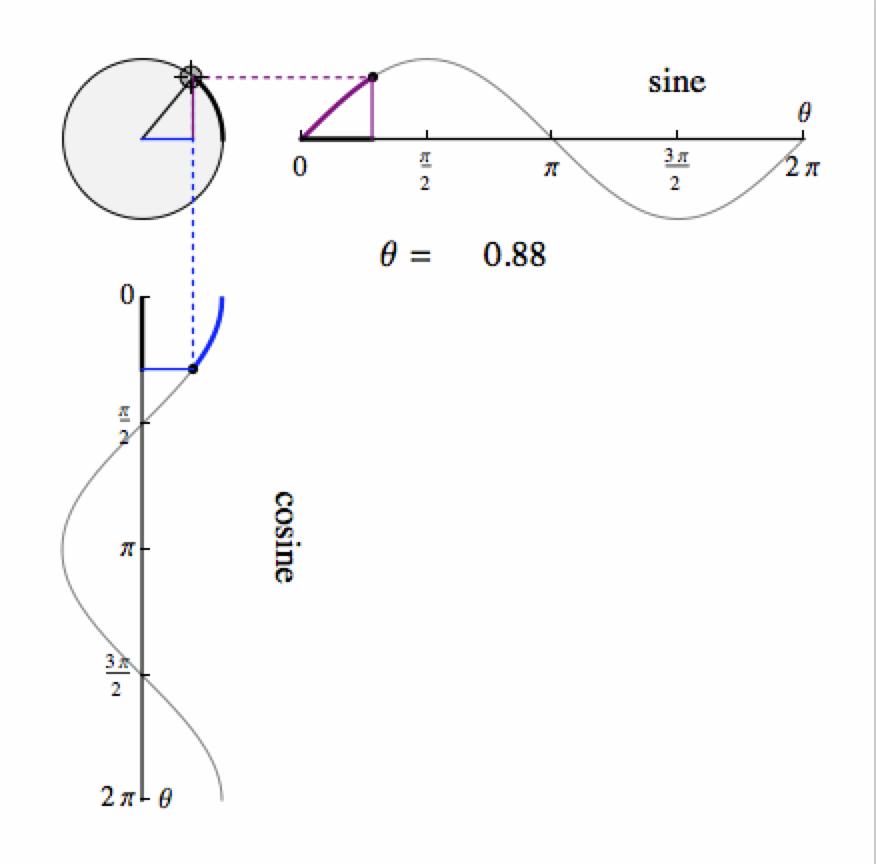The basic formulae here all reply on the SINE graph as follows:

• The sine of an angle is defined by the vertical height of a point as it rotates around a unit circle (that is, its radius is 1) measured from a horizontal line through the centre of the circle. So it cannot be bigger than 1 or less than -1.
• the cosine of an angle is defined by the horizontal distance of a point as it rotates around the unit circle measured from a vertical line through the centre of the circle. It too must be in the range -1 to 1.
• From the diagram it is easy to see that the sine and cosine graphs are the same shape, but displaced by a quarter turn (90°). In mathematical terms, we would say cosine(x) = sine(90°+x).
• The tangent of an angle measures the ratio of sine to cosine and so can take any value whatsoever.
At multiples of 90° it becomes infinite or negatively infinite.
Click on the diagram to go to the Mathematica Demonstrations website for the free Computable Document Format (CDF) version that plays in a free CDF Player and makes the animation live.
The Spinning Out Sine and Cosine Demonstration is by B Atwood and S Wagon.

The angles can be measured by several types of unit.

• Often this is degrees with a complete turn divided into 360 degrees
• If we define sine and cosine by distances (or coordinates) of a point on a unit circle, we can also define the angle by a distance on that circle too: the distance on the circumference that a point travels in turing through that angle. The circumference of a unit circle is 2 π. This measure is called a radian.
Here is a converter to convert between the two:

## Patterns

### The Simple Square-Root pattern

Ernesto La Orden of Madrid pointed out the following neat way to connect and remember the easiest of the sines (cosines):
00
 √4 =  1 2
90π
2
30π
6
 √3 2
60π
3
45π
4
 √2 = 1 2 √2
45π
4
60π
3
 √1 = 1 2 2
30 π
6
90π
2
 √0 = 0 2
00

### The √2 ± √n pattern

Ernesto La Orden also put many angles into this pattern:
cosine
sine
π
2
90°
 √2 – √4
=  02
0
5π
12
75°
 √2 – √32
15
3π
8
67.5°
 √2 – √22
22.5
π
3
60°
 √2 – √1
=  122
30
π
4
45°
 √2 – √0
=  12√2
45
π
6
30°
 √2 + √1
=  √322
60
π
8
22.5°
 √2 + √22
67.5
π
12
15°
 √2 + √32
75
0
 √2 + √4
=  12
90

### The √2 ± Phi pattern

cosine
sine
2π
5
72°\color{blue}{frac{\sqrt{2 - \Phi}}{2}}18°
3π
10
54°\color{blue}{\frac{\sqrt{2 - \varphi}}{2}36°
π
5
36°\color{blue}{\frac{\sqrt{2 + \varphi}}{2}}54°
π
10
18°\color{blue}{\frac{\sqrt{2 + \Phi}}{2}72°
\color{blue}{\Phi = \frac{\sqrt{5} + 1}{2} = 1.618033... = 1 + \varphi}
\color{blue}{\varphi = \frac{\sqrt{5} - 1}{2} = 0.618033.. = \Phi - 1}
\color{blue}{\Phi^2 = \frac{3+\sqrt{5}}{2} = 2.618033..}
\color{blue}{\varphi^2 = \frac{3 - \sqrt{5}}{2} = 0.381966..}

### The Golden Ratio

We can extend the previous pattern to include many angles which are simple fractions of π if we use the the golden ratio values Phi ($\Phi$) and phi ($\varphi$):
 cos(9°= π/20) = \color{blue}{ \frac{1}{2} \sqrt{2 + \sqrt{2 + \Phi}} = sin(81°) cos(18°=π/10) = \color{blue}{\frac{1}{2} \sqrt{2 + \sqrt{2 + \varphi}} = \frac{1}{2}\sqrt{2 + \Phi}} = sin(72°) cos(27°=3π/20) = \color{blue}{\frac{1}{2} \sqrt{2 + \sqrt{2 - \varphi}}} = sin(63°) cos(36°=π/5) = \color{blue}{\frac{1}{2} \sqrt{2 + \sqrt{2 - \Phi}} = \frac{1}{2}\sqrt{2 + \varphi} = \frac{\Phi}{2}} = sin(54°) cos(45°=π/4) = \color{blue}{\frac{1}{2} \sqrt{2 \pm \sqrt{2 - 2}} = \frac{\sqrt{2}}{2}} = sin(45°) cos(54°=3π/10) = \color{blue}{\frac{1}{2} \sqrt{2 - \sqrt{2 - \Phi}} = \frac{1}{2}\sqrt{2 - \varphi}} = sin(36°) cos(63°=7π/20) = \color{blue}{\frac{1}{2} \sqrt{2 - \sqrt{2 - \varphi}}} = sin(27°) cos(72°=2π/5) = \color{blue}{\frac{1}{2} \sqrt{2 - \sqrt{2 + \varphi}} = \frac{1}{2}\sqrt{2 - \Phi} = \frac{\varphi}{2}} = sin(18°) cos(81°=9π/20) = \color{blue}{ \frac{1}{2} \sqrt{2 - \sqrt{2 + \Phi}}} = sin(9°)
This pattern uses the identities
\color{blue}{phi = \sqrt{2 - \Phi}} and \color{blue}{\Phi = \sqrt{2 + \varphi}}

### A more general pattern

There is a more encompassing pattern here if we use all the following values under the innermost square-root:
\color{blue}{ 2, \sqrt{3}, \Phi, 1, \varphi, 0, -\varphi, -1, -\sqrt{3}, -\Phi, -2}
or
2, 1.732..., 1.618..., 1, 0.618..., 0, -0.618..., -1, -1.618..., -1.732..., -2
together with the half-angle formula for cos(A/2) (see below) starting from cos(36)=Phi/2 and cos(72)=phi/2. The pattern continues with the cosines of 4.5°, 13.5°, etc. and it includes the following angles which are fractions of π too:
 cos(0) = \color{blue}{\frac{1}{2} \sqrt{2 + \sqrt{2 + 2}} = 1} sin(90°) cos(7.5°=π/24) = \color{blue}{\frac{1}{2} \sqrt{2 + \sqrt{2 + \sqrt{3}}}} sin(82.5°) cos(37.5°=5π/24) = \color{blue}{\frac{1}{2} \sqrt{2 + \sqrt{2 - \sqrt{3}}}} sin(52.5°) cos(45°=π/4) = \color{blue}{\frac{1}{2} \sqrt{2 \pm \sqrt{2 - 2}} = \frac{\sqrt{2}}{2}} sin(45°) cos(52.5°=7π/24) = \color{blue}{\frac{1}{2} \sqrt{2 - \sqrt{2 - \sqrt{3}}}} sin(37.5°) cos(82.5°=11π/24) = \color{blue}{\frac{1}{2} \sqrt{2 - \sqrt{2 + \sqrt{3}}}} sin(7.5°) cos(90°=π/2) = \color{blue}{\frac{1}{2} \sqrt{2 - \sqrt{2 + 2}} = 0} sin(0°)

## Proofs

### 30° 45° and 60°

Here are two simple triangles which give us the formulae for the trig values of these three angles:-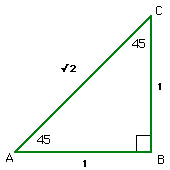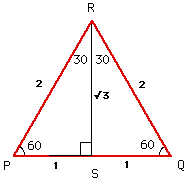This triangle is just a square cut along a diagonal. If the sides are of length 1, the diagonal is length √2. This gives the sin, cos and tan of 45°. Here is an equilateral triangle where all sides and all angles are equal (to 60°). If the sides are of length 2, then when we cut it in half as shown, the two triangles have 60°, 30° and 90° angles with a side of length 1 and a hypotenuse of length 2. The other side is therefore of length √3. So we can read off the sin cos and tan of both 30° and 60°.

### 36° and 54°, 18° and 72°For 36° and 72° we need some further work based on the geometry of a regular pentagon which has angles of 36° and 72°. If the sides of the pentagon are of length 1, the diagonals are of the golden section number in length Phi where:
 Phi =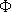= 1.618033988.. = 1 + √5 = 1 + 12Phi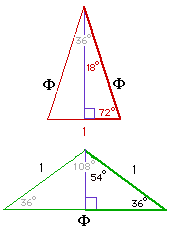The upper triangle with angles 72°, 72° and 36° and sides of lengths 1, Phi and Phi shows the trig values for 18° and 72°.  The lower triangle with angles of 36°, 36° and 108° and sides of lengths 1, 1 and Phi shows the trig values of 36° and 54°.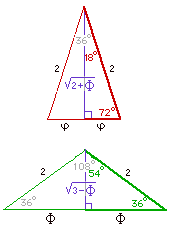### 15° and 75°

If we take the triangle on the left, we can calculate the length of the third side using the Cosine Formula. If, in a triangle with sides a, b and c, we know both sides b and c and also the angle A between sides b and c then we can compute the length of third side, a, as follows: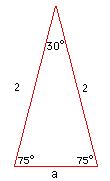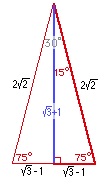a2 = b2 + c2 – 2 b c cos(A) For our triangle on the left, the known sides are b=2 and c=2 and the angle between them is A=30°. The length of the third side, the base a, is therefore: a2 = 22 + 22 – 2 x 2 x 2 x cos(30°) = 8 – 4 √3 = 2 (4 – 2 √3) But (√3 – 1)2 = 3 + 1 – 2 √3 = 4 – 2 √3 and so a2 = 2 (√3 – 1)2 Taking the square-root: a = √2 (√3 – 1) which we can also write as = 2 (√3 – 1) / √2 Using this expressions for a, we can expand the triangle by a factor of √2, to get rid of the denominator. Finally, we put in a line from the top of the triangle to the centre of the base a to make two right-angled triangles. This will halve the side a and cut the triangle into two and gets rid of the factor 2 also. We then arrive at the triangle on the right which shows the sines and cosines of 75° and 15°:

### Ailles Rectangle for 15° and 75°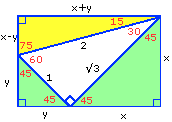An alternative (easier) method for sine and cosine of 15° and 75° is found in Ailles Rectangle (named after an Ontario high school teacher). It is easy to remember because it is two green 45° right-angled triangles stuck onto the sides of a white 30°-60°-90° triangle and the rectangle completed with a yellow 15°-75°-90° triangle on the hypotenuse of the 30°-60°-90° triangle as shown here.
The 30°-60°-90° sides are "as usual", namely 1, 2 and √3. From the two 45°-45°-90° triangles, it is quite easy to see that x is \color{blue}{sqrt 3 / sqrt 2} and y is \color{blue}{1/sqrt 2} from which we can read off the sines and cosines of 15° and 75°. However we will get some nicer numbers on the triangles if we expand all sides by a factor of √2. Click on the buttons underneath the image to see the (expanded) sizes.

## Trig Formulae

Many symmetries and patterns are apparent in the table. They reflect some underlying identities such as: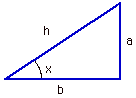sin(x) = a / h   cos(x) = b / h "cosec"(x) = h/a  sec(x)=h/b tan(x) = a / b cot(x) = b / a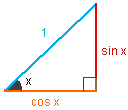\color{blue}{sin(x) = cos(90° - x)} \color{blue}{sin^2(x) + cos^2(x) = 1} \color{blue}{tan(x) = sin(x)/cos(x)} \color{blue}{cot(x) = 1/tan(x) = cos(x)/sin(x)} \color{blue}{tan^2(x) + 1 = 1/(cos^2(x))} \color{blue}{cot^2(x) + 1 = 1/(sin^2(x))}

If we know the value of a trig function on two angles a and b, we can determine the trig function values of their sum and difference using the following identities:

 sin(a+b)=sin(a)cos(b)+cos(a)sin(b) cos(a+b)=cos(a)cos(b)-sin(a)sin(b) \color{blue}{tan(a+b)=(tan(a)+tan(b))/(1-tan(a)tan(b))} sin(a-b)=sin(a)cos(b)-cos(a)sin(b) cos(a-b)=cos(a)cos(b)+sin(a)sin(b) \color{blue}{tan(a-b)=(tan(a)-tan(b))/(1+tan(a)tan(b))}

If the two angles are the same (i.e. a=b) we get the sines and cosines of double the angle. Rearranging those formulae gives the formula for the sin or cosine of half an angle:

 \color{blue}{sin(2a)} \color{blue}{=2sin(a)cos(a)} \color{blue}{sin(a/2)=sqrt((1-cos(a))/2)} \color{blue}{cos(2a) \color{blue}{=1-2sin^2(a)}  \color{blue}{cos(a/2)=sqrt((1+cos(a))/2)} \color{blue}{ = cos^2(a)-sin^2(a)} \color{blue}{=2 cos^2(a)-1} \color{blue}{2 csc(2a) = tan(a) + cot(a)} \color{blue}{tan(a/2) = (1-cos(a))/sin(a) = sin(a)/(1+cos(a))}

The following change a product of trig functions into a sum or difference and vice-versa:
 \color{blue}{sin(a) + sin(b) } \color{blue}{= 2 cos((a-b)/2) sin((a+b)/2)} cos(a) + cos(b)  \color{blue}{= 2 cos((a-b)/2) cos((a+b)/2)} sin(a) - sin(b)  \color{blue}{= 2 sin((a-b)/2) cos((a+b)/2)} cos(b) - cos(a)  \color{blue}{= 2 sin((a-b)/2) sin((a+b)/2)}

## A diagram to relate many angles and Phi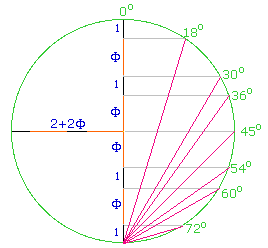Robert Gray's page on Coordinates for many regular solids has an amazing diagram at the bottom which relates Phi to the angles of 18°, 30°, 36°, 45°, 54°, 60° and 72° according to their 3D coordinates in the solids.

Each of those angles is measured from the top most point of the circle when a vertical line is turned through that angle.
Each line from the base point meets the circle at a point whose a height is 1 (72°), 1+Phi (60°), 2+Phi (54°), 2+2 Phi (45°), 2+3 Phi (36°), 3+3 Phi (30°) or 3+4 Phi (18°).

Do look at his pages for more fascinating information on 120 3D solids, of which we will also explore the most symmetrical 5 on our next page.

### You do the maths...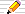1. Suppose the origin of the circle is (0,0) and its radius is 2 + 2 Phi. Find the equation of the circle.
2. Use your answer to the previous question to find the coordinates of each of the points on the circle with the angles shown.
3. Compute the lengths of each of the red lines from the lowest point to the points shown on the circle.
4.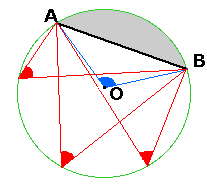From any two points A and B on a circle, the angle AOB at the centre of a circle, O, is twice the angle at any point on the circumference in the same sector.

In the diagram,all the red angles at the circumference are equal;
the red angles are half the blue angle AOB at the centre;
the red angles are to a point in the same sector of the circle as is the centre of the circle so they cannot be in the grey sector.

Use the above theorem to find three points on Robert Gray's circle immediately before this "You Do The Maths..." section where a line from the centre makes an angle with the vertical of
1. 2×18=36°
2. 2×30=60°
3. 2×36=72°

## Other angles with exact trig expressions involving square-roots

Are there other angles with a simple exact expression for their cosine or sine?
Well it all depends upon what you mean by simple!

Carl Friedrich GAUSS (177 - 1855) looked at a similar problem which answers this question. He investigated if there was a method of constructing a regular polygon of n sides using only a pair of compasses (to draw circles) and a straight-edge (a ruler with no markings). We know we can construct a regular polygon for all of the values of n=3, 4, 5, 6, 8 and 10.

### Halving

There is a simple geometrical way to use compasses to divide an angle into two (angle bisection). So all the angles in a regular n-gon can be split into two to make a regular 2n-gon. We can repeat the process to get a 4n-gon, 8n-gon and in general a 2kn-gon for any k once we have a method of constructing a regular n-gon.

The Trig Formula section above contains a formula for the cosine of half an angle in terms of the cosine of the (whole) angle:

\color{blue}{ cos(A/2) = sqrt ((1 +cos(A))/2) = (sqrt (2 + 2 cos(A)))/2}
As Mitch Wyatt pointed out to me, since we know that cos(90°) is 0 and 90° is π/2 radians, we can use it to find the cosine of half that angle (45° or π/4 radians) and then halve that angle again and so on. Each time we introduce another square root so we get a cascading or nested sequence of square roots:
\color{blue}{cos(pi/4)} = \color{blue}{(sqrt 2)/2}
\color{blue}{cos(pi/8)} = \color{blue}{(sqrt (2+sqrt 2))/2}
\color{blue}{cos(pi/16)} = \color{blue}{(sqrt(2+sqrt (2+sqrt 2)))/2}
\color{blue}{cos(pi/32)} = \color{blue}{(sqrt(2+sqrt(2+sqrt (2+sqrt 2))))/2}
However, this page is about sines and cosines which have simpler expressions, so we will not expand on this except to say that it shows how we can always find an exact expression for the sine (or cosine) of 1/2, 1/4, 1/8, ... ,1/2^n of any angle for which we have an exact sine (or cosine) expression.

### Superimposing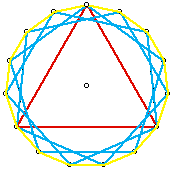If we construct a regular triangle (3 sides) and with the same circle centre, construct three regular pentagons (5 sides) with each having one vertex in common with the triangle, we will have the 15 vertices of a regular 15-gon.
This is shown on the right with the 3 pentagons in blue on the same circle, each having a vertex in common with the red triangle and the regular 15-gon appears in yellow.
By superimposing two regular polygons like this, we can construct a regular P×Q-gon (if P and Q have no factors in common otherwise more than one vertex of each will coincide).

### Do we know all the angles?

All this was known in Euclid's time, around the year 300 BC. So what about 7ths and 9ths? Is it possible to find sines and cosines of all the multiples of 1/7 and 1/9 of a turn in exact terms (using square roots)? What about 11ths and 12ths etc.?

In the next 2000 years no one found an exact geometric method for 7-gons or 9-gons but also no one had proved it was impossible to construct such regular polygons.

Then C F Gauss completely solved the problem while he was a student at Göttingen between 1795 and 1798. Gauss found the conditions on n and its prime factors to solve two equivalent problems:

• drawing a regular n-sided polygon using only a straight edge and compass and
• expressing the cos and sin of 360/n° using only square roots.
If we factor n as 2ap1bp2c..., i.e. a, b, c, ... are the powers of the prime factors of n: 2, p1, p2, ... (the prime's power is 0 if it is not a factor of n) then both of the problems are solvable when
• b,c,... and all the powers except a , the power of 2, must be 1, and
• the primes>2 that are factors of n (that is p1, p2, ...) must be of the form 22k+1 for some number k.
Both problems are solvable for these values of n and only for these values.

Prime numbers of the form 22k+1 are called Fermat primes. The series of numbers of the form 22k+1 begins

220 + 1 = 3, 221 + 1 = 5, 222 + 1 = 17, 223 + 1 = 257, 224 + 1 = 65537, ...
However not every number of the form 22k + 1 is prime -- and it is only the prime ones that we must have as factors of n.
The next one, 225 + 1 is 4294967297 and has a factor of 641 so it is not prime. In fact, we do not know if there are any more primes of this form except the first 5 listed above.

Such numbers, n, of the form Gauss describes are as follows, one per row, each a product of some of the Fermat primes (but each prime at most once) followed by its multiples of two. For any number in the table, its double is also in the table:

 2 4 8 16 32 64 128 256 ... 3 6 12 24 48 96 192 ... 5 10 20 40 80 160 ... 3×5= 15 30 60 120 240 ... 17 34 68 136 272 ... 3×17= 51 102 204 408 ... 5×17= 85 170 340 680 ... 3×5×17= 255 510 1020 2040 ... 257 514 1028 2056 ...
When put in order, we have
(1), 2, 3, 4, 5, 6, 8, 10, 12, 15, 16, 17, 20, 24, 30, 32, 34, 40, 48, 51, 60, 64, 68, 80, 85, 96, 102...
which is Sloane's A003401.

The odd terms (the left hand column apart from 2) is the series

1, 3, 5, 15, 17, 51, 85, 255, 257, 771, 1285, 3855, 4369, 13107, 21845, 65535, 65537, 196611, 327685, 983055, 1114129, 3342387, 5570645, 16711935, 16843009, 50529027, 84215045, 252645135, 286331153, 858993459, 1431655765, 4294967295
which is A045544 since we do not know any more Fermat primes beyond the fifth (65537).

Some interesting facts about these odd numbers (we include 1 here too) are:

• all these 31 odd numbers are divisors of 225–1 = 232 – 1 = 4294967295 (see A004729)
When written in binary they are all palindromic (are exactly the same when reversed).
If we continue the pattern, irrespective of whether 22k–1 is prime or not, what we find is the pattern of the Sierpinski Triangle. This is a fractal - smaller versions of itself are contained in all the larger versions. One way to form it is to picture the parity (the oddness and evenness) of the numbers in Pascal's Triangle where every odd number in Pascal's triangle is represented by a black square and every even number by a white one:
 1 = 12 3 = 112 5 = 1012 15 = 11112 17 = 100012 51 = 1100112 85 = 10101012 255 = 111111112
 1 1 1 1 2 1 1 3 3 1 1 4 6 4 1 1 5 10 10 5 1 1 6 15 20 15 6 1 1 7 21 35 35 21 7 1the odd numbers
 8 16 32 64 128 rows
In Pascal's Triangle, we can start from a top row of 0's (white) with a single element of 1 (odd, black) in it.
Then for the rows below we can use the rule that
every element is generated by adding together the two elements on the row above:
the one directly above and the one to the right.
 1 4+ 6 4 1 ↓ 1 5 10 10 5 1
In the diagrams here we only want an element's parity (whether it is odd or even), so we have the simple rule that
if both the elements on the row above are the same colour:
both black (odd) or both white (even)
the element will be white (even)
if the two elements on the row above are different
one odd, one even
the element is black (odd)
 odd odd even even even even
 odd even even odd odd odd

### The π/17 formula

The other small denominator fraction that we have not covered yet is the one Gauss proved had a formula involving square-roots, for the cosines and sines of multiples of $\frac{\pi}{17}$ radians.
The formulae, generated and simplified using Mathematica® are still quite complicated. For instance here is $cos(\frac{\pi}{17})$
 \color{blue}{cos(pi/17)}  \color{blue}{= sqrt(15+sqrt 17 + sqrt(34 - 2 sqrt 17) + sqrt(68+12 sqrt 17 - 4 sqrt(170 + 38 sqrt 17)))/(4 sqrt(2))} \color{blue}{= 0.98297309968390177828194884485...}
The multiples of this angle also involve variations of the same 4 expressions that we see here. Each of the four forms, A, B, C, D, is the sum or difference of the same two terms.
We will use a subscript of + or - as an abbrevation for the sign used in each variation. Here they all are:
 \color{blue}{A_(+) = 15 + sqrt(17)} \color{blue}{A_(-) = 15 - sqrt(17)} \color{blue}{B_(+) = sqrt(2) sqrt(17+sqrt(17))} \color{blue}{B_(-) = sqrt(2) sqrt(17 - sqrt(17))} \color{blue}{C_(+) = 17 + 3 sqrt(17)} \color{blue}{C_(-) = 17 - 3 sqrt(17)} \color{blue}{D_(+) = sqrt(2) sqrt(85+19 sqrt(17))} \color{blue}{D_(-) = sqrt(2)sqrt(85 - 19 sqrt(17))}
The expressions for the cosines and signs of multiples of angles π/17 = 33.2639222145..° up to 90° = π/2 radians are as follows:
a √32 cos2(a)
color{blue}{pi/17} color{blue}{A_(+)+B_(+)+2 sqrt(C_(+)-D_(+))}
color{blue}{{2 pi}/17} color{blue}{A_(+)-B_(-)+2 sqrt(C_(+)+D_(+))}
color{blue}{{3 pi}/17} color{blue}{A_(-)+B_(+)+2 sqrt(C_(-)+D_(-))}
color{blue}{{4 pi}/{17}} color{blue}{A_(+)+B_(-)-2 sqrt(C_(+)-D_(+))}
color{blue}{{5 pi}/{17} } color{blue}{A_(-)+B_(+)-2 sqrt(C_(-)+D_(-))}
color{blue}{{6\pi}/{17} } color{blue}{A_(-)-B_(+)+2 sqrt(C_(-)-D_(-))}
color{blue}{{7\pi}/{17} } color{blue}{A_(-)-B_(+)-2 sqrt(C_(-)-D_(-))}
color{blue}{{8\pi}/{17}} \color{blue}{A_(+)-B_(-)-2 sqrt(C_(+)+D_(+))}
Phew!

The next value with an odd denominator is π/257 and the exact expression for cos(π/257) using square-roots only will be even more complicated!

Tom Ace pointed out that there is more about this in chapter 15 of Oystein Ore's Number Theory and Its History from 1948 and now available as a Dover book (1988).

### The π/15 formula

If
 color{blue}{A_(+)=9+sqrt 5} color{blue}{A_(-)=9 - sqrt 5} color{blue}{B_(+)=sqrt(6(5+sqrt 5))} color{blue}{B_(-)=sqrt(6(5-sqrt 5))}
then we have
a 4cos2(a)
π
15
color{blue}{A_(+)+B_(-)}

15
color{blue}{A_(-)+B_(+)}

15
color{blue}{A_(+)-B_(-)}

15
color{blue}{A_(-)-B_(+)}

## A Trig Calculator using Decimal numbers of any length

Here is a Calculatorthat will let you check all the above results and more to as many decimal places as you like as well as evaluating expressions and finding continued fractions.
For example, to check the \color{blue}{cos(pi/17)} formula above type
into the Expression to Evaluate input box and then press the conversion button ( ) and get its decimal value which is 0.

For other values, such as \color{blue}{cos(pi/8)}, type in and evaluate it first as a decimal and then as a continued fraction showing that the CF is [0; 1, 12, 7, 3, 2, 1, 1, 1, 1, 20, 5, 3, 11, 1, 7, 7, 3,...]. Even looking ahead for many more terms there is no pattern here.
However, if we change the Expression to Evaluate to the CF this time is [0; 1, 5, 1, 4, 1, 4, 1, 4, 1, 4, 1, 4,...].
There is a clear pattern here that goes on for many terms. If we delete the 1,4,1,4,... part and put it into the recurring part input for the CF so that you have the CF [0; 1, 5, 1, 4] and then pressing the convert-to-fraction-form button () will show \color{blue}{(2+sqrt(2))/4} as the value of cos(pi/8)^2 and so we deduce that (computationally) \color{blue}{cos(pi/8)=sqrt(2+sqrt(2))/2}.

In this way we can experimentally discover many such expressions for trig forms using the ability of this Calculator to quickly evaluate to many decimal places and to (accurately and exactly) convert continued fractions to the form (a\pm sqrt(b))/c. Then we can look for a mathematical proof that our results are indeed true.
Change the Working Precision to 50, 100, or even 200 dps to see if patterns persist with more computational accuracy.

## References

• 66.41 Some Trigonometric Ratios in Surd Form J M H Peters The Mathematical Gazette Vol. 66, (1982), pages 296-299
though there are some errors in his formulae involving τ (our Phi) which are corrected in the equations on this page.

Back to the Fascinating Flat Phi Facts page
Back to the Continued Fractions page
© 1996-2017 Dr Ron Knott